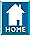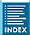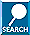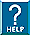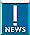VIMOS Quality Control:
astrometry
 HOME INDEX SEARCH HELP NEWS

QUALITY CONTROL     HOME CAL | HC | refs | QCVIMOS QC HOMEgeneral infotrending & QC1biasdarkdetlinscrflatskyflatzeropointssky2CCDimage quallampsMOS waveMOS efficMOS mask2CCDIFU calibIFU efficfeatures & problemsVIMOS Health Checksgeneral infoscience datascience recipecalib datacalib recipesproduct namesIFU componentsgeneral infoVIMOS infogeneral infoQC homeData ProductscalCheckerHealthChecksReference FramesQC1 databaseParanal autrep database(ESO internal) [best viewing with style sheets and javascript enabled]QC PLOTS CURRENT HISTORY Xccd - Xsky for Q1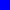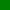Xccd - Xsky for Q2Xccd - Xsky for Q3Xccd - Xsky for Q4Yccd - Ysky for Q1Yccd - Ysky for Q2Yccd - Ysky for Q3Yccd - Ysky for Q4QC1 database (advanced users): browse | plot Click on CURRENT to see the current trending (Health Check).    Click on HISTORY to see the historical evolution of the trending.

VIMOS astrometric fields are typically observed once or twice each month. They are needed to provide the sky-to-CCD transformation matrix. The fields that are normally used are named 'Region E', 'Region M', and Region 'N'. Monitoring of the transformation coefficients is a prerequisite for enabling preimage-less MOS observations (PILMOS).

 top Sky to CCD transformation coefficients

The WCS can be used to transform celestial coordinates onto a virtual plane that is free of instrument distortions. The sky-to-CCD transformation converts these virtual coordinates into real (pixel) coordinates on the detectors. The transformation matrix has the following form:

Xccd = sum [ a_ij * X^j * Y^i ],
Yccd = sum [ b_ij * X^j * Y^i ], with i, j = 1, ..., m.

The pipeline recipe vmskyccd is executed with m=3. Only the constant and linear coefficients are used for the QC parameters, i.e.:

Xccd = a_00 + a_01 * X + a_10 * Y
Yccd = b_00 + b_01 * X + b_10 * Y .

QC1 parameters

 parameter QC1 database: table, name procedure xrms vimos_astrometry, sky_ccd_xrms rms of fit in x direction yrms vimos_astrometry, sky_ccd_yrms rms of fit in y direction a_00 vimos_astrometry, sky_ccd_x_0_0 a_00 coefficient of sky-to-CCD transformation a_10 vimos_astrometry, sky_ccd_x_1_0 a_10 coefficient of sky-to-CCD transformation a_01 vimos_astrometry, sky_ccd_x_0_1 a_01 coefficient of sky-to-CCD transformation b_00 vimos_astrometry, sky_ccd_y_0_0 b_00 coefficient of sky-to-CCD transformation b_10 vimos_astrometry, sky_ccd_y_1_0 b_10 coefficient of sky-to-CCD transformation b_01 vimos_astrometry, sky_ccd_y_0_1 b_01 coefficient of sky-to-CCD transformation relative distortion in x at (512,610) vimos_astrometry, delta_xsky_xccd_512_610 see below relative distortion in x at (1024,610) vimos_astrometry, delta_xsky_xccd_1024_610 see below relative distortion in x at (1536,610) vimos_astrometry, delta_xsky_xccd_1536_610 see below relative distortion in x at (512,1220) vimos_astrometry, delta_xsky_xccd_512_1220 see below overall shift in x at (1024,1220) vimos_astrometry, ref_xsky_xccd_1024_1220 see below relative distortion in x at (1536,1220) vimos_astrometry, delta_xsky_xccd_1536_1220 see below relative distortion in x at (512,1830) vimos_astrometry, delta_xsky_xccd_512_1830 see below relative distortion in x at (1024,1830) vimos_astrometry, delta_xsky_xccd_1024_1830 see below relative distortion in x at (1536,1830) vimos_astrometry, delta_xsky_xccd_1536_1830 see below relative distortion in y at (512,610) vimos_astrometry, delta_ysky_xccd_512_610 see below relative distortion in y at (1024,610) vimos_astrometry, delta_ysky_xccd_1024_610 see below relative distortion in y at (1536,610) vimos_astrometry, delta_ysky_xccd_1536_610 see below relative distortion in y at (512,1220) vimos_astrometry, delta_xsky_xccd_512_1220 see below overall shift in y at (1024,1220) vimos_astrometry, ref_xsky_xccd_1024_1220 see below relative distortion in y at (1536,1220) vimos_astrometry, delta_xsky_xccd_1536_1220 see below relative distortion in y at (512,1830) vimos_astrometry, delta_xsky_xccd_512_1830 see below relative distortion in y at (1024,1830) vimos_astrometry, delta_xsky_xccd_1024_1830 see below relative distortion in y at (1536,1830) vimos_astrometry, delta_xsky_xccd_1536_1830 see below

For monitoring the astrometric quality, nine virtual data points (X, Y) = (512, 610), (1024, 610), (1536, 610), (512, 1220), (1024, 1220), (1536, 1220), (512, 1830), (1024, 1830), (1536, 1830) are converted into CCD coordinates (Xccd, Yccd). The QC1 parameters ref_xsky_xccd_1024_1220 and ref_ysky_yccd_1024_1220 contain the difference between both, i.e. ref_xsky_xccd_1024_1220 = Xccd - X for (X, Y) = (1024, 1220). For the other parameters, this central difference is subtracted, e.g. delta_xsky_xccd_512_610 = Xccd - X - ref_xsky_xccd_1024_1220 for (X, Y) = (512, 610). This allows to distinguish between the overall shift of the transformation (measured by ref_xsky_xccd_1024_1220, etc.) and changes in the distortion (measured by delta_xsky_xccd_512_610, etc.).

History

 date event 2012-07 implementation of HC for astrometrySend comments to   Last update: September 6, 2013